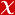## Time-Space Fractional Governing Equations of Unsteady Open Channel Flow

JOURNAL OF HYDROLOGIC ENGINEERING, vol.22, no.2, 2017 (SCI-Expanded)#### Abstract

In this study, the complete governing equations for unsteady open channel flow in fractional time-space are developed from the fractional continuity equation combined with the fractional motion equation, which is based on Newton's second law of motion, by accounting for the acceleration terms in order to render physically interpretable hydraulic terms. Then the kinematic wave and diffusion wave approximations to unsteady open channel flow with physically interpretable terms in fractional time-space are developed from the fractional continuity and motion equations. Because the powers of all of the derivatives in the conventional governing equations of unsteady open channel flow in integer time-space are one, these conventional equations are derived as special cases of the fractional governing equations when the fractional powers in the fractional equations approach unity.